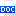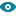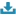# Giáo án Tiếng Anh 6 - Units 1: Greetings - Lesson 4: How old are you? (c1, 2)

I. Aim:

By the end of the lesson, students can count and use the cardinal numbers from 1 to 20.

II. Language contents:

1. Grammar: - one and two is three.

- three minus two is one.

2. Vocabulary:

- cardinal numbers from 1 to 20

- and, minus

III. Techniques:

• Game ( Lucky numbers)

• Slap the board

• Missing letters

• Pelmanism

IV. Teaching aids:

cards, cassette, tape2 trangvuhuy12325/10/20181260
Bạn đang xem tài liệu "Giáo án Tiếng Anh 6 - Units 1: Greetings - Lesson 4: How old are you? (c1, 2)", để tải tài liệu gốc về máy bạn click vào nút DOWNLOAD ở trên
```Lesson 4. HOW OLD ARE YOU? (C1, 2)
Date:
Period: 5
I. Aim:
By the end of the lesson, students can count and use the cardinal numbers from 1 to 20.
II. Language contents:
1. Grammar: - one and two is three.
- three minus two is one.
2. Vocabulary:
- cardinal numbers from 1 to 20
- and, minus
III. Techniques:
Game ( Lucky numbers)
Slap the board
Missing letters
Pelmanism
IV. Teaching aids:
cards, cassette, tape
V. Procedure:
Teacher’s and Students’ activities
Content
-T. gives words with missing letters.
Ss work in small groups to write the meaningful words.
Then they give feedback
- T. corrects.
- T. has Ss listen to some cardinal numbers from 1 to 20.
- T. presents new words, and guides Ss to read them aloud (choral, individual)
- T. corrects their pronunciation.
- T. has Ss play a game “Slap the board”.
Ss work in 2 groups.
- T. gives her idea.
- T. has Ss count cardinal numbers from 1 to 20 with a partner. (silently, aloud)
- Then T. has Ss play a game “Lucky numbers”.
Ss work in 2 groups.
- T. gives her idea.
- T. gives an exercise in Math.
Ss do in small groups, then they give feedback
- T. corrects their mistakes.
- T. has Ss play a game “Pelmanism”.
Ss work in 2 groups.
- T. gives her idea.
1. Warm up:
* Missing letters:
Go_d mo_nin_	Ho_ _re y_u?
G_od b_e	 I _m _ine, th_nks
T_ank y_u	 Ho_ _re y_u?
_hil_ren	 W_ are _ine, th_nks
2. Presentation:
* Cardinal numbers: 1 → 20
- one
- two
- three
- four
- five
- six
- seven
- eight
- nine
- ten
- eleven
- twelve
- thirteen
- fourteen
- fifteen
- sixteen
- seventeen
- eighteen
- nineteen
- twenty
- one and two is three.
- three minus two is one.
* Slap the board
20
7
8
15
1
10
19
16
4
17
3. Practice:
* Count from 1 to 20 with a partner.
* Lucky numbers
1	2	3	4
5	6	7	8
Lucky numbers: 2, 7
1. Count from 20 to 0
2. Lucky number
3. Count numbers of students in a group.
4. Rearrange this word “etevsneen”
5. Answer this question “How are you?”
6. Count even numbers from 0 to 20
7. Lucky number
8. Count odd numbers from 0 to 20
4. Production:
* Calculating:
* Marks:
a. 8 + 5 =
b. 16 - 6 =
c. 20 : 4 =
d. 6 x 3 =
e. four x four =
f. fifteen + two =
g. ten : two =
h. eight - seven =
* Pelmanism:
one
1
two
3
2
three
4
four
5. Homework:
- Learn by heart new words.
- Count cardinal numbers from 1 to 20 again.
- Prepare Unit 1. Lesson 5. C3, 4, 5
* new words
* pattern to ask and answer about age, introduce someone.
* Comments:
--------------------------------------------------------------------------------------------------------------------------------------------------------------------------------------------------------------------------------------------------
-------------------------------------------------------------------------------------------------------------------------
```

Tài liệu đính kèm:

•Unit_1_Greetings.doc